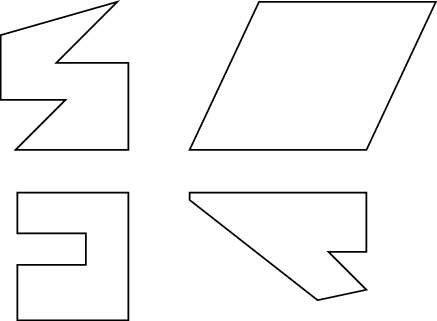##### Basic Math & Pre-Algebra For Dummies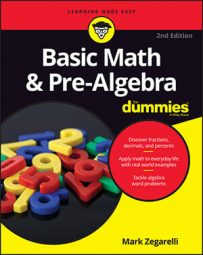A polygon is any shape whose sides are all straight. Every polygon has three or more sides (if it had fewer than three, it wouldn't really be a shape at all). Following are a few of the most common polygons.

## Triangles

The most basic shape with straight sides is the triangle, a three-sided polygon. You find out all about triangles when you study trigonometry. Triangles are classified on the basis of their sides and angles. Take a look at the differences:
• Equilateral: An equilateral triangle has three sides that are all the same length and three angles that all measure 60 degrees.

• Isosceles: An isosceles triangle has two sides that are the same length and two equal angles.

• Scalene: Scalene triangles have three sides that are all different lengths and three angles that are all unequal.

• Right: A right triangle has one right angle. It may be isosceles or scalene.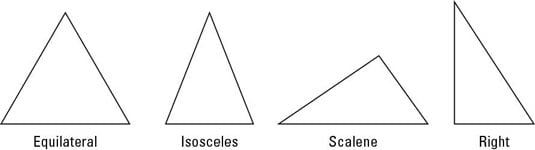A quadrilateral is any shape that has four straight sides. Quadrilaterals are one of the most common shapes you see in daily life. If you doubt this statement, look around and notice that most rooms, doors, windows, and tabletops are quadrilaterals. Here are a few common quadrilaterals:
• Square: A square has four right angles and four sides of equal length; also, both pairs of opposite sides (sides directly across from each other) are parallel.

• Rectangle: Like a square, a rectangle has four right angles and two pairs of opposite sides that are parallel. Unlike the square, however, although opposite sides are equal in length, sides that share a corner — adjacent sides — may have different lengths.

• Rhombus: Imagine starting with a square and collapsing it as if its corners were hinges. This shape is called a rhombus. All four sides are equal in length, and both pairs of opposite sides are parallel.

• Parallelogram: Imagine starting with a rectangle and collapsing it as if the corners were hinges. This shape is a parallelogram — both pairs of opposite sides are equal in length, and both pairs of opposite sides are parallel.

• Trapezoid: The trapezoid's only important feature is that at least two opposite sides are parallel.

• Kite: A kite is a quadrilateral with two pairs of adjacent sides that are the same length.

• Isosceles trapezoid: A trapezoid in which the nonparallel sides (the legs) are congruent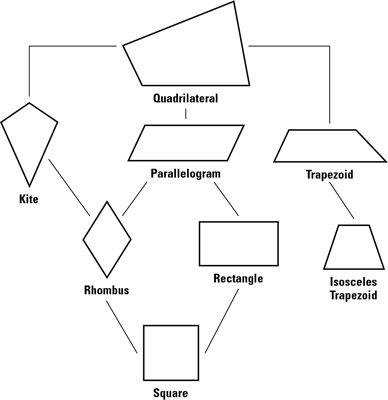In the hierarchy of quadrilaterals shown in the above figure, a quadrilateral below another on the family tree is a special case of the one above it. A rectangle, for example, is a special case of a parallelogram. Thus, you can say that a rectangle is a parallelogram but not that a parallelogram is a rectangle (a parallelogram is only sometimes a rectangle).

## Polygons on steroids — larger polygons

A polygon can have any number of sides. Polygons with more than four sides aren't as common as triangles and quadrilaterals, but they're still worth knowing about. Larger polygons come in two basic varieties: regular and irregular.

A regular polygon has equal sides and equal angles. The most common are regular pentagons (five sides), regular hexagons (six sides), and regular octagons (eight sides).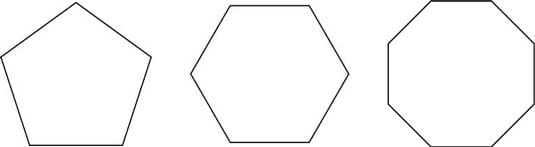Every other polygon is an irregular polygon.